# Oops...

This website is made for modern browsers. You are seeing this, because your browser is missing a feature or two. Please install the latest update or switch to a modern browser. See you soon.

# Truss grid

In this tutorial, we will create a truss grid. For this, we need a basic grid pattern and trussed girders along the axes of the grid. Meanwhile, we learn common operations with data trees

.

## Grasshopper

#### 1

##### Generate a basic grid pattern

First thing to do, is to create the basic grid. For this, we use a Square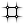Square (SqGrid)
Vector  >  Grid  >  Square
2D grid with square cells
Inputs
Plane (P)Base plane for grid
Size (S)Size of grid cells
Extent X (Ex)Number of grid cells in base plane x direction
Extent Y (Ey)Number of grid cells in base plane y direction
Outputs
Cells (C)Grid cell outlines
Points (P)Points at grid corners
component and, at the moment, we are interested in the inputs S (Size), Ex (Extent X), and Ey (Extent Y). By connecting a Number Sliders to these inputs, we can dynamically control the size and extend of the square grid.

Now, lets change the slider output to whole numbers: After creating a Number Slider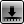Number Slider
Params  >  Input  >  Number Slider
Numeric slider for single values
on the canvas, it’s initialized with the default values, which are floating-point numbers. To change their format, we can double-click the slider on its left side (on the name, not the scale) and a menu opens; here we can adjust the settings. In this case, we change the Rounding to N (Integer Numbers) and set the Numeric domain to a Min of 5 and a Max of 10. Now the slider will return whole numbers within the set range. We connect this slider to input S.

Another way to create a slider that returns integers in a given range, is to use the Canvas search and type 2..20. After pressing Enter a slider is placed on the canvas that ranges from 2 to 20 and we connect it to the inputs Ex and Ey.

#### 2

##### Create the vertical members

After generating the basic grid, we get its corner points at output P. To create the vertical members, we need to create a second set of points above our basic grid. For this, we use a Move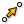Move (Move)
Transform  >  Euclidean  >  Move
Translate (move) an object along a vector.
Inputs
Geometry (G)Base geometry
Motion (T)Translation vector
Outputs
Geometry (G)Translated geometry
Transform (X)Transformation data
component and connect the corner points to its input G, which collects the geometry we want to move. Input T takes the translation vector.

The translation vector is defined with a Unit Z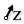Unit Z (Z)
Vector  >  Vector  >  Unit Z
Unit vector parallel to the world {z} axis.
Inputs
Factor (F)Unit multiplication
Outputs
Unit vector (V)World {z} vector
component. This gives us the direction of the vector. To set the vector length, we adjust the unit multiplication factor at input F with another slider. After typing 0.5..1.5 into the Canvas Search, we get a Number SliderNumber Slider
Params  >  Input  >  Number Slider
Numeric slider for single values
with once decimal ranging from 0.5 to 1.5. After wiring the components, we can now dynamically change the offset of the second set of points.

To create the vertical members, we use a Line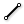Line (Ln)
Curve  >  Primitive  >  Line
Create a line between two points.
Inputs
Start Point (A)Line start point
End Point (B)Line end point
Outputs
Line (L)Line segment
component and connect the points at ground level to input A and the moved points to input B.

#### 3

##### Create the upper and lower chords

At output P of the grid, the points at the grid corners are structured in a data tree format

: each branch holds the points for one column. If we connect a PolyLine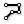PolyLine (PLine)
Curve  >  Spline  >  PolyLine
Create a polyline connecting a number of points.
Inputs
Vertices (V)Polyline vertex points
Closed (C)Close polyline
Outputs
Polyline (Pl)Resulting polyline
component to these points, we get polylines in the column direction.

To create lines in the row direction, we need to change the structure of the data tree by using a Flip Matrix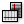Flip Matrix (Flip)
Sets  >  Tree  >  Flip Matrix
Flip a matrix-like data tree by swapping rows and columns.
Inputs
Data (D)Data matrix to flip
Outputs
Data (D)Flipped data matrix
component. Now each branch holds the points for one row. Thus, another PolyLinePolyLine (PLine)
Curve  >  Spline  >  PolyLine
Create a polyline connecting a number of points.
Inputs
Vertices (V)Polyline vertex points
Closed (C)Close polyline
Outputs
Polyline (Pl)Resulting polyline
component will create lines in the row direction.

The just created polylines represent the lower chords of our truss grid and we need another MoveMove (Move)
Transform  >  Euclidean  >  Move
Translate (move) an object along a vector.
Inputs
Geometry (G)Base geometry
Motion (T)Translation vector
Outputs
Geometry (G)Translated geometry
Transform (X)Transformation data
component to create the upper chords.
Because the geometry we feed into a Move component is still available in the component(s) before, we use Move for copying something (and there is no Copy component). We just need to remember that if we want to progress the chords, we have to connect wires to the outputs off all three components to grab all chords.
Because the geometry we feed into a Move component is still available in the component(s) before, we use Move for copying something (and there is no Copy component). We just need to remember that if we want to progress the chords, we have to connect wires to the outputs off all three components to grab all chords. We can connect two wires with input G by holding down Shift while connecting the wires. At input T we connect the translation vector from step 2.

#### 4

##### Generate a sub grid

The next thing we want to create are the diagonal members. Here, we don’t want the diagonals to run between the points from before, but in a zigzag with a higher frequency (as shown in the sketch).

To find the points for the higher frequency, we will use another SquareSquare (SqGrid)
Vector  >  Grid  >  Square
2D grid with square cells
Inputs
Plane (P)Base plane for grid
Size (S)Size of grid cells
Extent X (Ex)Number of grid cells in base plane x direction
Extent Y (Ey)Number of grid cells in base plane y direction
Outputs
Cells (C)Grid cell outlines
Points (P)Points at grid corners
grid with smaller cells. Because the sub grid is depending on the main grid, we have to use the initial sliders to keep the algorithm parametric. In this example, we will place four diagonals between the main grid corners. Therefore, we use a Division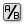Division (A/B)
Maths  >  Operators  >  Division
Mathematical division
Inputs
A (A)Item to divide (dividend)
B (B)Item to divide with (divisor)
Outputs
Result (R)The result of the Division
component and connect the Size slider to input A. At input B we connect a PanelPanel
Params  >  Input  >  Panel
A panel for custom notes and text values
with the value of 4. Now 4 cells of the sub grid span the distance of one cell from the main grid.

Because the cells of the sub grid are smaller, we need more of them to cover the area of the main grid: We use a Multiplication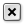Multiplication (A×B)
Maths  >  Operators  >  Multiplication
Mathematical multiplication
Inputs
A (A)First item for multiplication
B (B)Second item for multiplication
Outputs
Result (R)Result of multiplication
component and connect it to the Extend slider and also to the Panel with 4.

#### 5

##### Identify the needed points from the sub grid

The output P returns all the points at the sub grid corners, however, we only need those that are on the axes of the main grid. We could either find them by analyzing their geometric location or by selecting the desired branches by name. In this example, we retrieve the points by doing the latter. To get the names of the branches, we hook up a Tree Statistics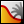Tree Statistics (TStat)
Sets  >  Tree  >  Tree Statistics
Get some statistics regarding a data tree.
Inputs
Tree (T)Data Tree to analyze
Outputs
Paths (P)All the paths of the tree
Length (L)The length of each branch in the tree
Count (C)Number of paths and branches in the tree
component.

The names of the branches can be found at output P. Now, we need every fourth branch (or to be more precise, the first, then the fifth etc). To get every fourth name, we use a Partition List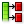Partition List (Partition)
Sets  >  List  >  Partition List
Partition a list into sub-lists
Inputs
List (L)List to partition
Size (S)Size of partitions
Outputs
Chunks (C)List chunks
component and connect the names to input L and the panel with 4 to input S. This will chop the list of names into branches with four items each. Connecting a List Item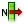List Item (Item)
Sets  >  List  >  List Item
Retrieve a specific item from a list.
Inputs
List (L)Base list
Index (i)Item index
Wrap (W)Wrap index to list bounds
Outputs
Item (i)Item at {i'}
component with default values will return the first item of each branch and those are the names we need.

#### 6

##### Extract the needed sub points

Now that we have the names for the branches we want, we use a Tree Branch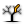Tree Branch (Branch)
Sets  >  Tree  >  Tree Branch
Retrieve a specific branch from a data tree.
Inputs
Tree (T)Data Tree
Path (P)Data tree branch path
Outputs
Branch (B)Branch at {P}
component and connect the points to input T and the names to input P. This will select the columns of the sub grid that are on the main grid.

We also need the rows of the sub grid that are on the main grid. To get them, we need to use a Flip MatrixFlip Matrix (Flip)
Sets  >  Tree  >  Flip Matrix
Flip a matrix-like data tree by swapping rows and columns.
Inputs
Data (D)Data matrix to flip
Outputs
Data (D)Flipped data matrix
component on the points before connecting them to another Tree BranchTree Branch (Branch)
Sets  >  Tree  >  Tree Branch
Retrieve a specific branch from a data tree.
Inputs
Tree (T)Data Tree
Path (P)Data tree branch path
Outputs
Branch (B)Branch at {P}
component.

#### 7

##### Move and pair the sub points

With the completion of step 6, we have the desired points from the sub grid. But, the column and row direction are separated in two components. We will now combine them into a single data tree, so that we can move the whole tree of points instead of two individual trees. To combine different trees into one tree, on which each branch contains an old tree, we will use the Entwine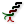Entwine (Entwine)
Sets  >  Tree  >  Entwine
Flatten and combine a collection of data streams
Inputs
Branch {0;0} ({0;0})Data to entwine
Branch {0;1} ({0;1})Data to entwine
Branch {0;2} ({0;2})Data to entwine
Outputs
Result (R)Entwined result
components. However, the default settings don’t offer what we are looking for and therefore, we right-click the component and deselect Flatten Inputs.

After connecting each direction of points to its own input, we zoom in until we see the zoomable user interface and then press the little minus to remove the spare input. Now, we use another MoveMove (Move)
Transform  >  Euclidean  >  Move
Translate (move) an object along a vector.
Inputs
Geometry (G)Base geometry
Motion (T)Translation vector
Outputs
Geometry (G)Translated geometry
Transform (X)Transformation data
component together with the translation vector from step 2 to create points on the upper chords.

Next, we want to combine the two sets of sub points into a data tree. But this time, we want branches that contain the two points that are on top of each other. Placing a Merge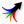Merge (Merge)
Sets  >  Tree  >  Merge
Merge a bunch of data streams
Inputs
Data 1 (D1)Data stream 1
Data 2 (D2)Data stream 2
Outputs
Result (R)Result of merge
component on the canvas and connecting the points from the upper and lower chords to it, will return lists of points that share the same column or row. To get the two opposing points in each list, we graft both inputs of Merge by right-clicking the input and selecting Graft.
See essentials/data-trees#graft
to learn how the data is restructured when grafting.
See essentials/data-trees#graft
to learn how the data is restructured when grafting.

#### 8

##### Flip every second pair of points

To run the zigzag for the diagonals, we will flip the order of every second pair of points. To select every second branch, we use a Split Tree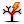Split Tree (Split)
Sets  >  Tree  >  Split Tree
Split a data tree into two parts using path masks.
Inputs
Data (D)Tree to split
Outputs
Positive (P)Positive set of data (all branches that match any of the masks)
Negative (N)Negative set of data (all branches that do not match any of the masks
component and connect the branches to input D. For input M, we create a PanelPanel
Params  >  Input  >  Panel
A panel for custom notes and text values
with the content {*;0,2,...}. This pattern will select all branches that end with in even number.
The how-to Split Tree
explains possible patterns in more detail.
The how-to Split Tree
explains possible patterns in more detail.
At output P we get the positive matches and at output N the negative ones.

To flip the order of the positive matches, we use a Reverse List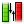Reverse List (Rev)
Sets  >  List  >  Reverse List
Reverse the order of a list.
Inputs
List (L)Base list
Outputs
List (L)Reversed list
component.
Alternatively, we could right-click an input or output grip and select Reverse in the context menu.
Alternatively, we could right-click an input or output grip and select Reverse in the context menu. Then, we combine both lists with another MergeMerge (Merge)
Sets  >  Tree  >  Merge
Merge a bunch of data streams
Inputs
Data 1 (D1)Data stream 1
Data 2 (D2)Data stream 2
Outputs
Result (R)Result of merge
component.

#### 9

##### Create the diagonal members

After step 8, we have lists in which the first item is alternately on the lower and upper chord. Now, we will create a polyline that connects every first point. To grab the first item from a list, we use List ItemList Item (Item)
Sets  >  List  >  List Item
Retrieve a specific item from a list.
Inputs
List (L)Base list
Index (i)Item index
Wrap (W)Wrap index to list bounds
Outputs
Item (i)Item at {i'}
. Now there is one item in each branch. To get all points in one column or row, we have to remove the last ramification with Trim TreeTrim Tree (Trim)
Sets  >  Tree  >  Trim Tree
Reduce the complexity of a tree by merging the outermost branches.
Inputs
Tree (T)Data tree to flatten
Depth (D)Number of outermost branches to merge
Outputs
Tree (T)Trimmed data tree
.

Finally, we connect a PolyLinePolyLine (PLine)
Curve  >  Spline  >  PolyLine
Create a polyline connecting a number of points.
Inputs
Vertices (V)Polyline vertex points
Closed (C)Close polyline
Outputs
Polyline (Pl)Resulting polyline
component to the output, which results in the generation of the diagonal members.

## Get the results

### Version Info

• Rhino 6.30
• Grasshopper 1.0.0007

Well, the above was certainly fun because the algorithm now includes all kinds of data tree operations, but of course, there is a quicker way, especially for creating the zigzag. We can, for example, not only use Split TreeSplit Tree (Split)
Sets  >  Tree  >  Split Tree
Split a data tree into two parts using path masks.
Inputs
Data (D)Tree to split
Outputs
Positive (P)Positive set of data (all branches that match any of the masks)
Negative (N)Negative set of data (all branches that do not match any of the masks
to get every second path, but also every fifth one. Also, we can use specialized components to Merge lists more efficiently to get the points we need for the zigzag. A good place to start is after step 4.

Hint 1

### Extract the needed sub points

As said, we can use Split Tree to select the branches we need from the sub gird. But, before we do that, we should use a Flip MatrixFlip Matrix (Flip)
Sets  >  Tree  >  Flip Matrix
Flip a matrix-like data tree by swapping rows and columns.
Inputs
Data (D)Data matrix to flip
Outputs
Data (D)Flipped data matrix
component to also get the other direction. Then we combine both trees into one with EntwineEntwine (Entwine)
Sets  >  Tree  >  Entwine
Flatten and combine a collection of data streams
Inputs
Branch {0;0} ({0;0})Data to entwine
Branch {0;1} ({0;1})Data to entwine
Branch {0;2} ({0;2})Data to entwine
Outputs
Result (R)Entwined result
(right-click to deselect Flatten Inputs).

Then we can use the Split TreeSplit Tree (Split)
Sets  >  Tree  >  Split Tree
Split a data tree into two parts using path masks.
Inputs
Data (D)Tree to split
Outputs
Positive (P)Positive set of data (all branches that match any of the masks)
Negative (N)Negative set of data (all branches that do not match any of the masks
component and set the pattern to {*;0,4,...}, which selects every fifth branch from the sub grid. Using MoveMove (Move)
Transform  >  Euclidean  >  Move
Translate (move) an object along a vector.
Inputs
Geometry (G)Base geometry
Motion (T)Translation vector
Outputs
Geometry (G)Translated geometry
Transform (X)Transformation data
with the translation vector from step 2 will create the second layer of points. Now we can mix and match them to create the zigzag. Just remember: each branch that we connect to the PolyLinePolyLine (PLine)
Curve  >  Spline  >  PolyLine
Create a polyline connecting a number of points.
Inputs
Vertices (V)Polyline vertex points
Closed (C)Close polyline
Outputs
Polyline (Pl)Resulting polyline
component to create the diagonals needs to have the same amount of points as after Split Tree.

Hint 2

### Combine the points

There are different ways to combine the points for creating the diagonal members. You can do it either way.

###### Pick’n’Choose

We could use Pick'n'Choose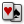Pick'n'Choose (P'n'C)
Sets  >  List  >  Pick'n'Choose
Pick and choose from a set of input data.
Inputs
Pattern (P)Pick pattern of input indices
Stream 0 (0)Input stream 0
Stream 1 (1)Input stream 1
Outputs
Result (R)Picked result
and connect the lower points to one input and the upper points to another. Then, we also need a pattern which picks points alternately. The pattern is defined in a PanelPanel
Params  >  Input  >  Panel
A panel for custom notes and text values
with two items; 1 and 0 (make sure to untoggle Multiline Data in the context menu of the panel).

Pick’n’Choose will not repeat the pattern internally and thus, we have to add a Repeat Data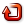Repeat Data (Repeat)
Sets  >  Sequence  >  Repeat Data
Repeat a pattern until it reaches a certain length.
Inputs
Data (D)Pattern to repeat
Length (L)Length of final pattern
Outputs
Data (D)Repeated data
component. The length of the pattern is given by the amount of points we have on one chord. This number can be retrieved with List Length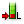List Length (Lng)
Sets  >  List  >  List Length
Measure the length of a list.
Inputs
List (L)Base list
Outputs
Length (L)Number of items in L
.

###### Weave and Dispatch

We could also use the Weave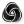Weave (Weave)
Sets  >  List  >  Weave
Weave a set of input data using a custom pattern.
Inputs
Pattern (P)Weave pattern of input indices
Stream 0 (0)Input stream 0
Stream 1 (1)Input stream 1
Outputs
Weave (W)Weave result
component and connect each set of chords to one input. Weave will create a list with twice the amount of points needed, placing the points from the lower and upper chords alternately: First point from the lower chord, opposing point on the upper chord, second point from the lower chord, opposing point on the upper chords, and so forth.

Given this pattern, we just need to grab the desired points, which we do with a Dispatch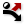Dispatch (Dispatch)
Sets  >  List  >  Dispatch
Dispatch the items in a list into two target lists.
Inputs
List (L)List to filter
Dispatch pattern (P)Dispatch pattern
Outputs
List A (A)Dispatch target for True values
List B (B)Dispatch target for False values
component. We can also set a pattern on this component and, in this case, the pattern needs to be 0, 1, 1, 0 (untoggle Multiline Data). Output A returns the remaining points that we are looking for.

###### Sift Pattern and Combine Data

Unlike with Weave, where we reduced the number of points after combining them, we can also reduce the unnecessary points before combining. We do this with Sift Pattern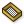Sift Pattern (Sift)
Sets  >  List  >  Sift Pattern
Sift elements in a list using a repeating index pattern.
Inputs
List (L)List to sift
Sift Pattern (P)Sifting pattern
Outputs
Output 0 (0)Output for sift index 0
Output 1 (1)Output for sift index 1
. As pattern, we use 1 and 0 again and connect it to one Sift Pattern for the lower chords and one for the upper chords.

To combine them, we use Combine Data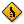Combine Data (Combine)
Sets  >  List  >  Combine Data
Combine non-null items out of several inputs
Inputs
Input 0 (0)Data to combine
Input 1 (1)Data to combine
Outputs
Result (R)Resulting data with as few nulls as possible
Index (I)Index of input that was copied into result
and connect output 0 to input 0 and vice versa to get the zigzag. If you inspect the lists, you notice that Sift Pattern will not delete the unnecessary items but replaces them with Null Items. Combine Data will then fill the null items with items from the other list.

Get the results

## Get the results

### Version Info

• Rhino 6.30
• Grasshopper 1.0.0007